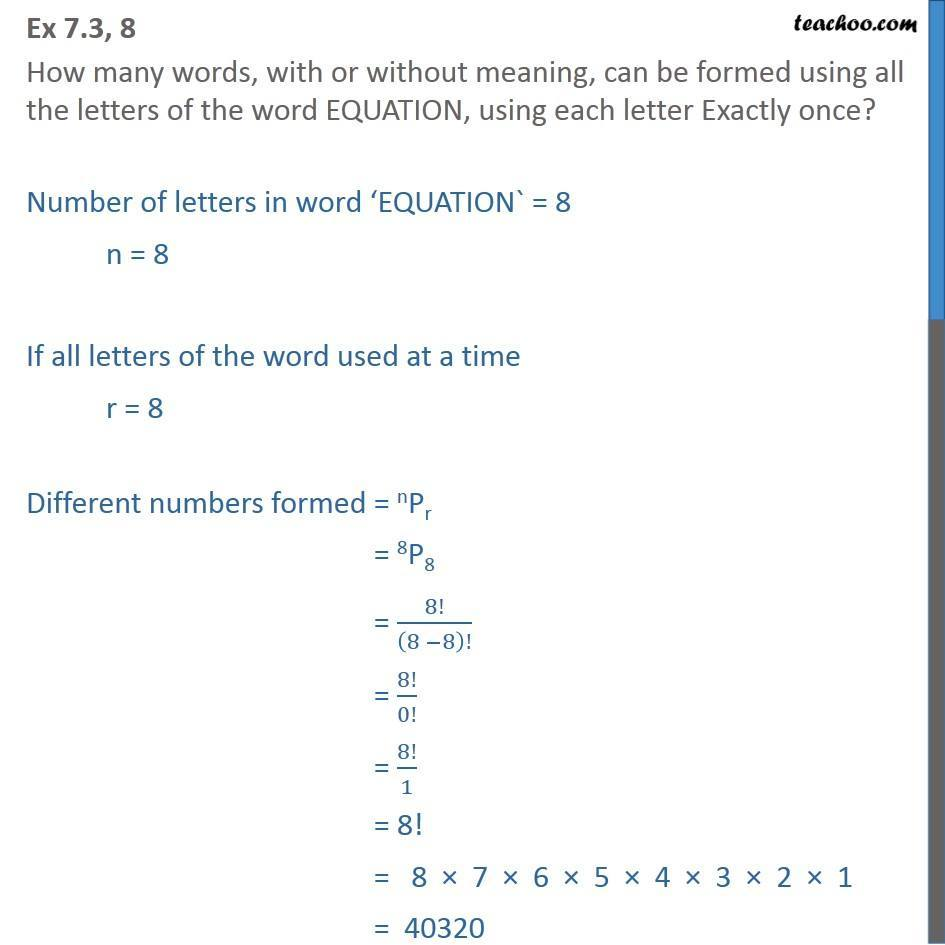1. Chapter 7 Class 11 Permutations and Combinations (Term 2)
2. Serial order wise
3. Ex 7.3

Transcript

Ex 7.3, 8 How many words, with or without meaning, can be formed using all the letters of the word EQUATION, using each letter Exactly once? Number of letters in word EQUATION` = 8 n = 8 If all letters of the word used at a time r = 8 Different numbers formed = nPr = 8P8 = 8!/(8 8)! = 8!/0! = 8!/1 = 8! = 8 7 6 5 4 3 2 1 = 40320